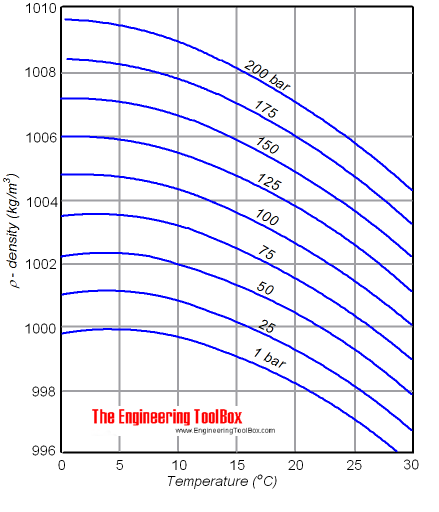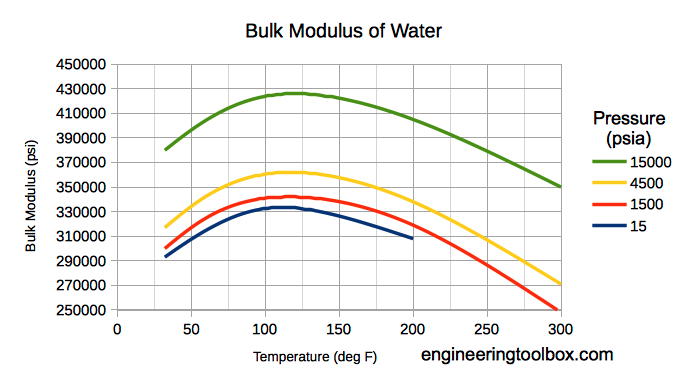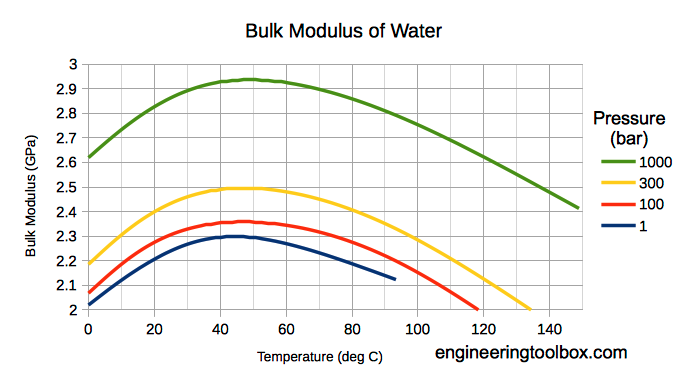Engineering ToolBox - Resources, Tools and Basic Information for Engineering and Design of Technical Applications!

# Liquids - Densities vs. Pressure and Temperature Change

## Densities and specific volume of liquids vs. pressure and temperature change.

The density of a liquid will change with temperature and pressure. The density of water versus temperature and pressure is indicated below:See also Water - Density, Specific Weight and Thermal Expantion Coefficient, for online calculator, figures and tables showing changes with temperature.

### Density

The density of a liquid can be expressed as

ρ = m / V                                (1)

where

ρ = density of liquid (kg/m3)

m = mass of the liquid (kg)

V = volume of the liquid (m3)

The inverse of density is specific volume:

v = 1 / ρ

= V / m                             (2)

where

v = specific volume (m3/kg)

### Volume and change in Temperature

When temperature increases -  most liquids expands:

dV = V1 - V0

= V0 β dt

= V0 β (t1 - t0)                               (3)

where

dV = V1 - V0 = change in volume - difference between final and initial volume (m3)

β = volumetric temperature expansion coefficient (m3/m3 oC)

dt = t1 - t0 = change in temperature - difference between final and initial temperature (oC)

(3) can be modified to

V1 = V0 (1 + β (t1 - t0))                           (3b)

### Density and change in Temperature

With (1) and (3b) the final density after a temperature change can be expressed as

ρ1 = m / (V0 (1 + β (t1 - t0)))                              (4)

where

ρ1 = final density (kg/m3)

- or combined with (2)

ρ1ρ0 / (1 + β (t1 - t0))                                  (4b)

where

ρ0 = initial density (kg/m3)

#### Volumetric Temperature Coefficients - β

Note! - volumetric temperature coefficients may vary strongly with temperature.

### Density and change in Pressure

The influence of pressure on the volume of a liquid can be expressed with the three dimensional Hooke's law

E = - dp / (dV / V0

= - (p1 - p0) / ((V1 - V0) / V0)                                   (5)

where

E = bulk modulus - liquid elasticity (N/m2)

The minus sign corresponds to the fact that an increase in the pressure leads to a decrease in volume.

With (5) - the final volume after pressure change can be expressed as

V1 = V0 (1 - (p1 - p0) / E)                                     (5b)

Combining (5b) with (1) - the final density can be expressed as:

ρ1 = m / (V0 (1 - (p1 - p0) / E))                                  (6)

- or combined with (2) - the final density can be expressed as

ρ1 = ρ0 / (1 - (p1 - p0) / E)                                  (6b)

#### Bulk Modulus Fluid Elasticity some common Fluids - E

• water : 2.15 109 (N/m2)
• ethyl alcohol : 1.06 109 (N/m2)
• oil : 1.5 109 (N/m2)

Note! Bulk modulus for liquids varies with pressure and temperature.

#### Bulk modulus for water - Imperial Units#### Bulk modulus for Water - SI units### Density of a fluid changing both Temperature and Pressure

The density of a fluid when changing both temperature and pressure can be expressed combining (4b) and (6b):

ρ1ρ1(from eq.1) / (1 - (p1 - p0) / E)

= ρ0 / (1 + β (t1 - t0)) / (1 - (p1 - p0) / E)                                (7)

### Example - Density of Water at 100 bar and 20oC

• density of water 0oC: 999.8 (kg/m3)
• expansion coefficient of water at 10oC: 0.000088 (m3/m3oC) (average value between 0 and 20oC)
• bulk modulus of water: 2.15 10(N/m2)

Density of water can be calculated with (3):

ρ1 = (999.8 kg/m3) / (1 + (0.000088 m3/m3oC) ((20 oC) - (0 oC))) / (1 - ((100 105 Pa) - (1 105 Pa)) / (2.15 109 N/m2)

= 1002.7 (kg/m3)

## Related Topics

• Fluid Mechanics - The study of fluids - liquids and gases. Involving velocity, pressure, density and temperature as functions of space and time.
• Thermodynamics - Work, heat and energy systems.
• Densities - Densities of solids, liquids and gases. Definitions and convertion calculators.

## Engineering ToolBox - SketchUp Extension - Online 3D modeling!

Add standard and customized parametric components - like flange beams, lumbers, piping, stairs and more - to your Sketchup model with the Engineering ToolBox - SketchUp Extension - enabled for use with the amazing, fun and free SketchUp Make and SketchUp Pro .Add the Engineering ToolBox extension to your SketchUp from the SketchUp Pro Sketchup Extension Warehouse!

Translate

## Privacy

We don't collect information from our users. Only emails and answers are saved in our archive. Cookies are only used in the browser to improve user experience.

Some of our calculators and applications let you save application data to your local computer. These applications will - due to browser restrictions - send data between your browser and our server. We don't save this data.

## Citation

• Engineering ToolBox, (2009). Liquids - Densities vs. Pressure and Temperature Change. [online] Available at: https://www.engineeringtoolbox.com/fluid-density-temperature-pressure-d_309.html [Accessed Day Mo. Year].

Modify access date.

. .

#### Scientific Online Calculator6 24